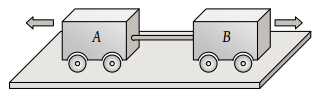Two carts of masses 200 kg and 300 kg on horizontal rails are pushed apart. Suppose the coefficient of friction between the carts and the rails are same. If the 200 kg cart travels a distance of 36 m and stops, then the distance travelled by the cart weighing 300 kg is(1) 32 m

(2) 24 m

(3) 16 m

(4) 12 m

Concept Videos :-

#16-Static-Friction-Force
#17-Solved-Examples-8
#18-Solved-Examples-9
#19-Solved-Examples-10
#20-Kinetic-Friction-Force
#21-Solved-Examples-11

Concept Questions :-

Friction

(3)

Let velocity of both carts be  just after the separation.

By conservation of momentum-

$\frac{{s}_{2}}{{s}_{1}}={\left(\frac{{m}_{1}}{{m}_{2}}\right)}^{2}={\left(\frac{200}{300}\right)}^{2}$

${s}_{2}={s}_{1}×\frac{4}{9}=36×\frac{4}{9}=16\text{\hspace{0.17em}}m$

Difficulty Level:

• 19%
• 35%
• 40%
• 7%